10.6  & 10.7  Physics experiment: simulation of moving electrons, hydrogen and boron ions in a magnetic field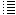Computer simulation of idea 10

Experiment 6 & experiment 7

 Experiment 6: B=10 gauss Generating 30 electrons, each electron with a kinetic energy of 100 eV Beamcurrent =  0,001 nA (in this way we get with only 30 electrons a nice circle) Generated  3 B+, starting with speed=0 above at (x=0,5s, y=0,5s, z=0,75s) and 3 H+ ions starting with speed=0 below at (x=0,5s, y=0,5s, z=0,25s), see fig. 1 Droomcomputersimulation.html  for coordinate system. After about 15 min computer time  all electrons are generated and moving in a nice circle. Now we increase the field to B=100 gauss. In the computer simulation this is easy to do, in reality ?? In the computer simulation we obtain a kind of pulsating circle, see video. The electrons seem to be not spreading out... Experiment 6 video (after 0,00056 sec, about 24 computer time hours later) (looks like not any difference, electrons do not spread out)

An intuitive way of explaining this:

First the electrons move in a (big) circle. When we increase the magnetic field, they start to move in smaller circles, see figure 1.

Fig. 1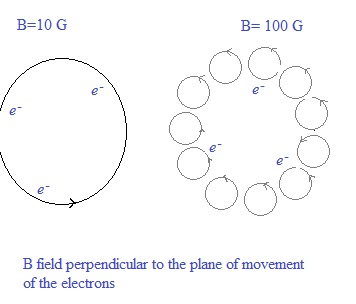Each electron moving in a small circle could be considered a small magnet.
What happens if an electron moves up or down, in vertical direction?
Perhaps the south poles get attracted by the north poles which prevent that they leave the plane in which they are moving, see figure 2.

Fig. 2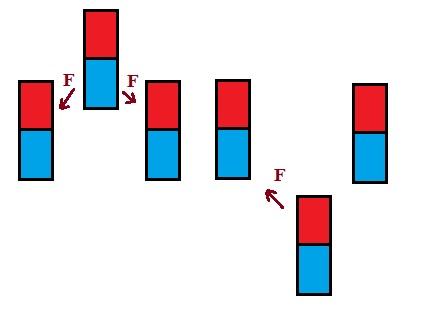But what about leaving in a horizontal direction?

Fig. 3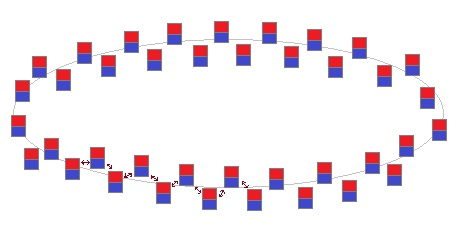If all the small magnets (electrons moving in a small circle) are alternately a little bit  upwards and downwards  positioned, then the forces between them will
prevent them both to move away up or down, and to move away in a horizontal direction.

Just trying to explain... Anyway, in the simulation, as already mentioned, they seem not to spread out....

Experiment 7:

In reality it will not be possible to change the magnetic field instantaneously, because, if we use an electromagnet,
it will have an selfinductance which will delay the building up of a new magnetic field..

After having increased  (slowly) the magnetic field, the situation would be as ilustrated in figure 4 (if I am not wrong...)

Fig. 4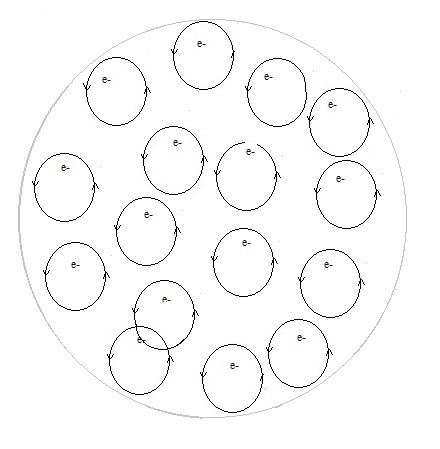Imagine an electron moving in the big circle. Then the B-field increases a little bit, so the electrons move a little out
of the big circle towards the centre, moving in a circle with a slightly smaller radius. Then the B-field increases again a little bit,
and the electrons will move in a new circle with again a slightly smaller radius etc.

Fig. 5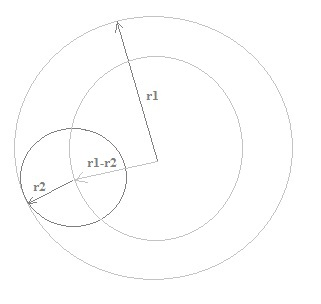First the electrons move in the big circle due to magnetic field B1 ->  r1= m.v /( q.B1)  (m= massa electron, v = speed electron, q = charge electron)

Then we increase the field to B2  ->  r2= m.v /( q.B2)

We will adapt the computer program: when the B field changes, we will distribute the electrons randomly inside the circle with radius r1-r2 .

Fig. 6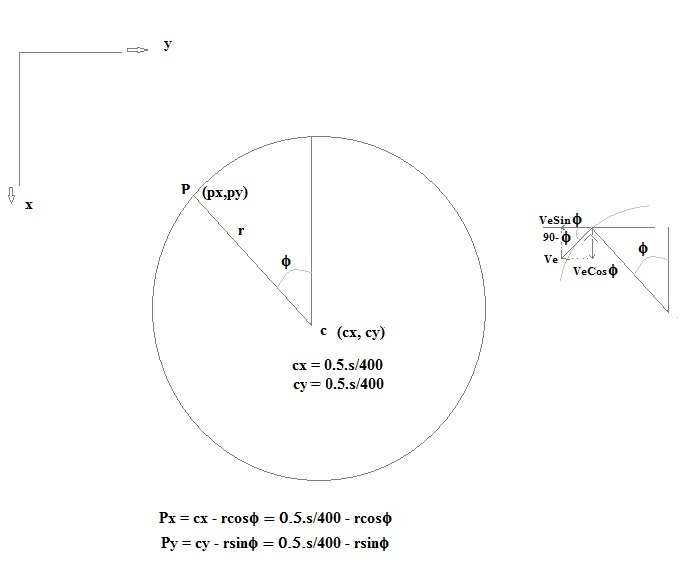Fig. 7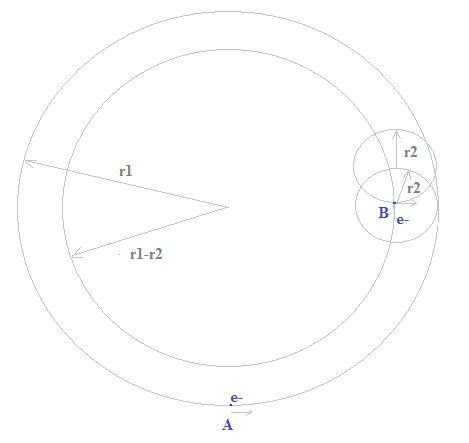In the program we choose r and f randomly. r = r1 - r2.  See fig. 5 & 6.  The field B can only be increased (otherwise an error in the program).

Each electron is thus distributed randomly inside the circle with radius r.

Not correct: the program could take the electron in point A and put it in point B, but the velocity stays the same. Then this electron could go (a little bit) outside the circle with radius r1, and in reality this can not happen. Every time the B increases, the electron will move in a circle with a smaller radius. Thus in a circle inside the former circle... and can never go outside the first circle.

Not correct: when the program randomly distributed the electrons, its electrical potential (Coulomb's law) could increase, but the total kinetic energy of the electrons stays constant.

Correct should be to change the magnetic field during, for example1 second, and let the program run. But the program runs so slowly, that we should have to wait 80 years...  (1000dt is about 1 second computer time if there are 50 electrons).

Anyway the program in experiment 7 seems to demonstrate that a collection of 50 electrons moving in small circles stay "together" in a  " simple " magnetic  field.

Remark:  the magnetic field in the centre of the cube is 1E2 gauss, and at the sides, top and bottom a little but larger (1,1E2 gauss).

 Experiment 7: 50 electrons, 100 eV, 0,001 nA, 3 B+, 3 H+, B=10 G, after about 30 min (just generated all 50 electrons), increased the field to B=100 G   After increasing the field, the electrons are randomly distributed inside the former circle (with radius=me*ve/(B*qe).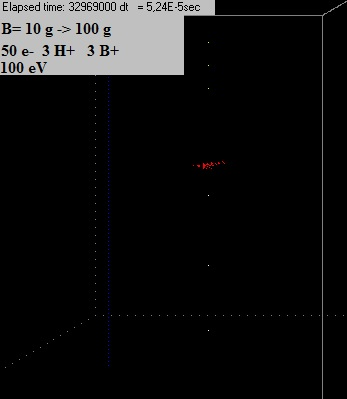Video experiment 7   (after 5,18E-5 s) After 5,18E-5 sec, about 8 hrs computer time, the electrons still did not spread out.. Video experiment 7 (after 0.000173 s) And after 0,000173 s neither.... Video experiment 7 (after 0.00036 s) A few electrons are now a bit farther away from the centre. Video experiment 7 (after 0.00037 s)  They came again a little bit closer to the centre. Video experiment 7 (after 0.000596 s, about 4,3 computertime days)  1 or 2 electrons have escaped out of the chamber. The rest are still ubicated in the centre (except a few that are somewhat away from the centre), but seemed to have slowed down and moving in small circles. In the program the total kinetic energy of the electrons cannot increase. If it increases a little bit  then the speed of each electron is multiplied with 0.999 in a repeat loop, until the present total kinetic energy of the electrons < initial total kinetic energy. But the total kinetic energy can yes decrease, and that is probable what has happened (due to the fact the this simulation is an approximation of reality, the same way you can approximate a smooth line by a stepwise drawn line... The program should be improved and the total kinetic and potential energy of all particles should remain constant minus the looses according the Larmor Formula. Video experiment 7b (after 8,27E-6 s)  The magnetic field has just been increased from 10 g to 100 g and  the electrons are randomly regrouped inside the first circle (see fig. 4). The total kinetic energy of all particles can now neither increase nor decrease (within very small limits). If it changes, then the speed each electron is multiplied by 0.999 or by 1.001 in a repeat loop, until it is again (almost) the same as the initial kinetic energy of all particles (there are only 3 H+ and 3 B+ ions, their speed is not altered, I do not think this is important). The Larmor Formula looses (about 0.1 % in 1 sec) will be neglected for the moment. Also it should be nice to see the data (speed, coorditates) of each particle. Now they can only be seen in the screen and sometimes it´s difficult to determine well their position and velocity.   In the former experiments we generated the electrons in a point of their orbiting circle inside the vacuum chamber. In reality not very handy to let the electron gun point inwards the vacuum chamber were fusion should take place. Let´s now see if it is possible to generated them in point (0,5s, s, 0,5s), in the middle of the right side, and let them again orbit in a circle around the centre of the cube... From experiment 3 the total kinetic energy of all particles can not increase (when it increases beyond a limit, the speed of the electrons is reduced a bit until it´s again under this limit). The kinetic energy can however yes decrease, and this is what possible happened in experiment 7. Now in experiment 9 it can neither decrease. Furthermore the potential energy is calculated .

1.10-9 A during 10 s  = 1.10-9 Q/s .10 s / ( 1,16.10-19 Q/s) = 8,6.1010 electrons. A lot more than in the program, and impossible to simulate with it...

 (Jan. 2013) (Jan. 2013) (Jan. 2013) (3 Febr. 2013) (6 Febr. 2013) (8 Febr. 2013) (16 Jan. 2014) (17 Jan. 2014) (20 Jan. 2014) ( Febr. 2014- 2016) Computer simulation tenth idea (2014-2016) Eleventh idea   (2016.. 2017)